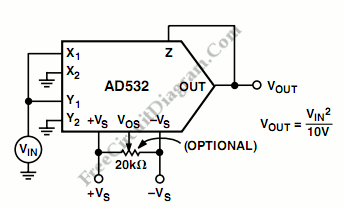## LT1016 – LS74121 Voltage Controlled Pulse Width Generator/ Monostable Multivibrator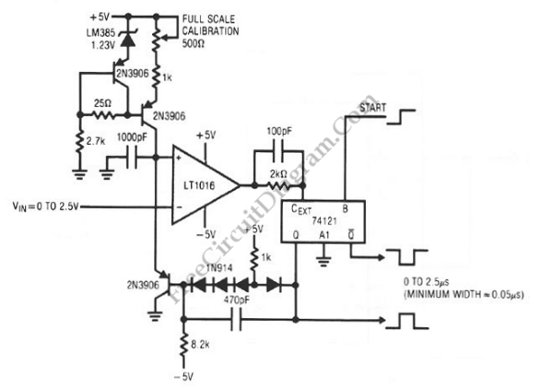Mono-stable multivibrator circuit generates a fixed pulse width when receiving a trigger signal on its input. The pulse width of the output is fixed regardless of the input pulse duration/width. In practical application, the width of the output pulse is determined by choosing the right components values for certain application. In today’s digital electronics, mono-stable function can be easily implemented […]

## Wien-Bridge Oscillator Using HA2541 Op-Amp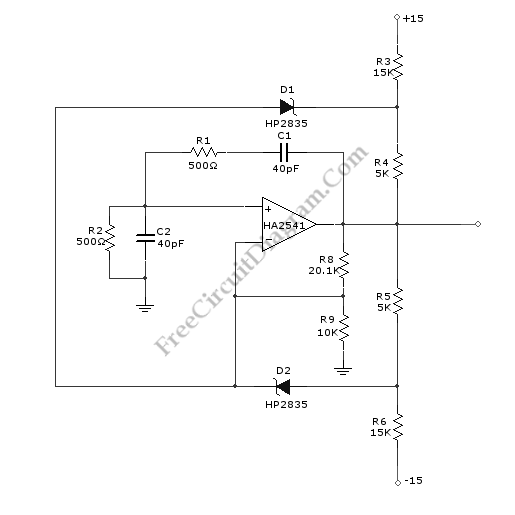A Wien-Bridge oscillator circuit can be built from HA2541 and some basic components. This circuit can generate good-quality sine wave of 40 MHz with an upper limit of 50 MHz. The R3 through R7 and D2 and D1 provide the diode limiting for this circuit. Here is the schematic diagram of this circuit: For frequency stability, this circuit need a […]

## Analog Multiplier-Divider with 4136 Op-Amps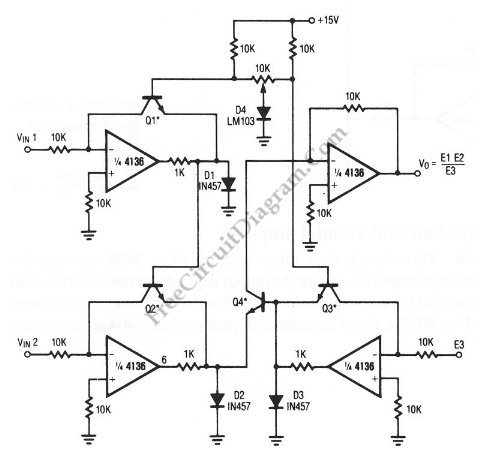This unique circuit below can be used as divider and multiplier. This circuit is formed by all four sections of a 4136 . The Q1 through Q4 can be any PNP transistor, but that must matched transistors. The transistor matching determine the acurracy of the divider and multiplier circuit. Here is the schematic diagram of the circuit:

## Square Root Mode for AD532 Analog Processor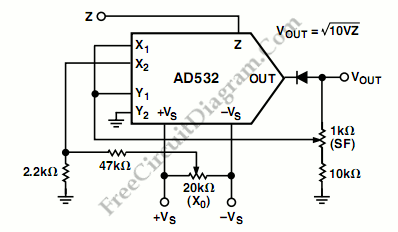The following schematic diagram shows us about the connections for square root mode for ADS532 analog processor chip.  Similar to the division mode, the multiplier cell is connected in the feedback of the op amp by connecting the output back to both the X and Y inputs. To prevent latch up as Zi approaches 0 Volts, the diode D1 is […]

## Basic Logic Gate with PNP Transistor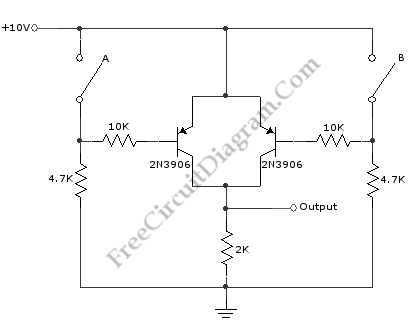This schematic diagram shows a circuit of basic logic gate circuit. This PNP transistor circuit can act as OR gate or AND gate. If the input is closing of switch, this circuit acts as AND gate. This circuit acts as OR gate when the input is opening of switch. The phase inversion of input does not complicate overall circuitry. Here […]

## Single Chip Divider Circuit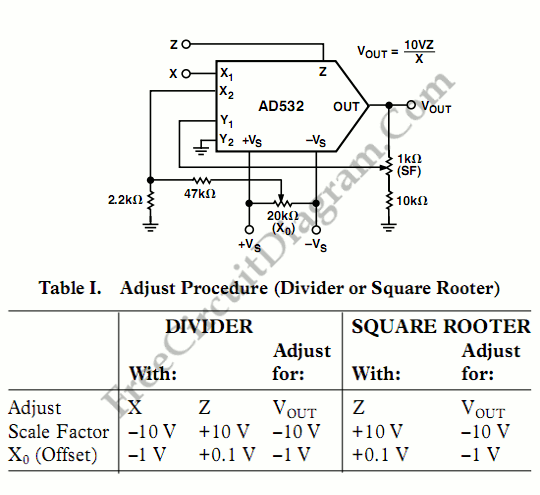We can build a divider circuit using only a single chip component, a special purpose integrated circuit AD532. We can configure the AD532 as a two quadrant divider by connecting the multiplier cell in the feedback loop of the op amp and using the Z terminal as a signal input, as shown in the following figure. For your note, the […]